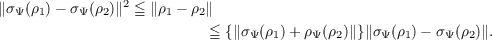#### Vol. 50, No. 2, 1974

 Download this articleFor screen For printingRecent Issues Vol. 325: 1 Vol. 324: 1  2 Vol. 323: 1  2 Vol. 322: 1  2 Vol. 321: 1  2 Vol. 320: 1  2 Vol. 319: 1  2 Vol. 318: 1  2Online Archive Volume: Issue:The Journal Subscriptions Editorial Board Officers Contacts Submission Guidelines Submission Form Policies for Authors ISSN: 1945-5844 (e-only) ISSN: 0030-8730 (print) Special Issues Author Index To Appear Other MSP Journals
Some properties of modular conjugation operator of von Neumann algebras and a non-commutative Radon-Nikodym theorem with a chain rule

### Huzihiro Araki

Vol. 50 (1974), No. 2, 309–354
##### Abstract

For a cyclic and separating vector Ψ of a von Neumann algebra R, the corresponding modular conjugation operator JΨ is characterized by the property that it is an antiunitary involution satisfying JΨΨ = Ψ,JΨRJΨ = Rand ,QjΨ(Q)Ψ) 0 for all Q R where jΨ(Q) = JΨQJΨ.

The strong closure V Ψ of the vectors QjΨ(Qis shown to be a JΨ-invariant pointed closed convex cone which algebraically span the Hilbert space H. Any JΨ-invariant Φ H has a unique decomposition Φ = Φ1 Φ2 such that Φj V Ψ and sR1) sR2).

There exists a unique bijective homeomorphism σΨ from the set of all normal linear functionals on R onto V Ψ such that the expectation functional by the vector σΨ(ρ) is ρ. It satisfiesAny two σΨ and σΨ are related by a unitary uin Rby uσΨ(ρ) = σΨ(ρ) for all ρ.

The relation 1 ρ2 holds if and only if there exists A(ρ2∕ρ1) R such that A(ρ2∕ρ1)σΨ(ρ1) = σΨ(ρ2). The smallest l is given by A(ρ2∕ρ1). It satisfies the chain rule A(ρ3∕ρ2)A(ρ2∕ρ1) = A(ρ3∕ρ1). It coincides with the positive square root of the measure theoretical Radon-Nikodym derivative if R is commutative.

As an application, it is shown that product of any two modular conjugation jΨjΦ is an inner automorphism of R.

For a product state ρj of a C algebra generated by finite W tensor products {⊗jIRj}⊗{⊗jI1j} of von Neumman algebras Rj, it is shown that ρj and ρj are equivalent if and only if ΣσΨ(ρj) σΨ(ρj)2 < where σΨ(ρ) σΨ(ρ)is independent of Ψ.

It is shown that there exists a unitary representation UΨ(g) of the group of all -automorphisms of R such that UΨ(g)αjUΨ(g) = g(x) for all x R and UΨ(g)σΨ(gρ) = σΨρ for all normal positive linear functionals ρ.

Primary: 46L10
##### Milestones